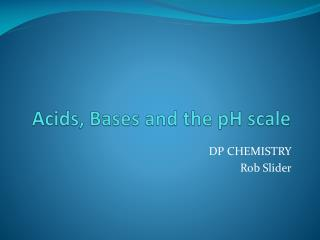DownloadDownload PresentationAcids, Bases and the pH scale

# Acids, Bases and the pH scale

Download Presentation## Acids, Bases and the pH scale

- - - - - - - - - - - - - - - - - - - - - - - - - - - E N D - - - - - - - - - - - - - - - - - - - - - - - - - - -
##### Presentation Transcript

1. Acids, Bases and the pH scale DP CHEMISTRY Rob Slider

2. ACIDS Acids are compounds that: • have a low pH (below 7) • taste sour • turn blue litmus paper red • react with bases to neutralize them and produce salts • release H2 gas in reactions with active metals • aqueous solutions conduct electricity • furnish H+ • burn the skin if strong • dissolve carbonates These are some common acids. What others can you think of? Make a list in your notes.

3. BASES Bases are compounds that: • have a high pH (above 7) • taste bitter • turn red litmus paper blue • react with acids to neutralize them and produce salts • are slippery feeling • aqueous solutions conduct electricity • burn skin if strong • react with fats to form soap • furnish OH- What other bases/alkalis do you know?

4. Common ACIDS Some common laboratory acids HCl hydrochloric acid HClO4perchloric acid HBrhydrobromic acid HNO3 nitric acid HI hydroiodic acid H2SO4 sulfuric acid Five of these acids are classified as monoprotic acids. They only have one hydrogen which they are able to donate. Sulfuric acid is classified as a diprotic acid because it has two acidic hydrogens that it can donate. Similarly, an acid which has three donatable hydrogens would be classified as triprotic.

5. Common BASES Some common laboratory bases NaOH sodium hydroxide Ba(OH)2 calcium hydroxide KOH potassium hydroxide Na2CO3 sodium carbonate NH3 ammonia NaHCO3 sodium hydrogen carbonate Bases are often found in everyday products such as many cleaning products (sodium hydroxide), antacid products (magnesium hydroxide )and fertilisers (ammonia). It is a common misconception that bases are not as dangerous as acids. In fact, many bases can be as much or more corrosive than many acids. Note that an alkali is a base that is soluble in water

6. Equilibrium constant of water Kw Note that water slightly dissociates in equilibrium with hydrogen and hydroxide ions: H2O  H+ + OH- So, the equilibrium constant is: Kw = [H+][OH-] Experimentally, it has been determined in a neutral solution at 250C: [H+] = [OH-] = 10-7 (a very small amount!) So, Kw = (10-7)(10-7) = 10-14 This shows how far to the left this equilibrium is

7. Neutralisation From the previous slide, Kw = [H+][OH-] Kw = (10-7)(10-7) = 10-14 This value (10-14) is constant for water so, addition of H+ means a decrease in [OH-] an increase in OH- means a decrease in [H+]

8. pH pH is a measure of the acidity of a solution. More accurately stated it is a measure of the hydrogen ion concentration on a logarithmic scale. pH stands for ‘potential of hydrogen’ Mathematically this is , pH = - log [H3O+] or pH = - log [H+] The inverse of the above expression is [H+] = 10-pH So, in a neutral solution, the concentration of hydrogen is 10-7 M: pH = -log[H+] = -log(10-7) pH = 7

9. The logarithmic scale The log scale is based on multiples of ten where the log is the value of exponent when the number is written as an exponent. To calculate pH, just use the log button on your calculator You try: If an acid has an H+ concentration of 0.0001 M, find the pH. Solution:First convert the number to exponential notation, find the log, then solve the pH equation. H+ = 0.0001M = 10-4; log of 10-4 = -4; pH = - log [ H+] = - log (10-4) = - (-4) = +4 = pH

10. pH scale The pH scale, (0 - 14), is the full set of pH numbers which indicate the concentration of H+ and OH- ions in water. pH Scale Principle: H+ ion concentration and pH relate inversely.OH- ion concentration and pH relate directly. The following statements may be made about the pH scale numbers. Complete the last twoa. Increasing pH means the H+ ions are decreasing.b. Decreasing pH means H+ ions are increasing.c. Increasing pH means OH- ions ared. Decreasing pH means OH- ions are

11. pH exercises

12. pH exercises (solutions)

13. pH of some common substances Notice that an increase in 1 pH value means a ten fold increase in hydrogen ion concentration

14. pH Indicators • Many substances change colour as they are exposed to different pH levels. These can be used to “indicate” the pH of substances when the colour ranges are known • Some natural products such as litmus, cabbage, grapes and tea are natural indicators while others such as phenolphthalein and methyl orange are synthetic • pH indicators are themselves acids or bases as they donate or accept protons Notice the variety of ranges where different indicators change colours. Some have more than one change.

15. pH Indicators Some specific examples: • Litmus red5 8blue • Phenolphthalein colourless8.3 10red • Methyl orange red3.1 4.4yellow • Bromothymol blue yellow6 7.6blue Note: colour changes within these ranges are gradual

16. A pH problem - indicators An unknown solution produces the colours above. What is the pH range of this solution? 8-8.3

17. pH meters A pH meter is used to accurately measure pH and takes the ‘guesswork’ out of judging colours using indicators. Basically, they work by very precisely measuring the voltage. This voltage is then converted to a pH value by the meter.